« Vrati se

#### IMO Shortlist 2010 problem G2

Let$P$ be a point interior to triangle$ABC$ (with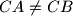$CA \neq CB$). The lines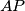$AP$,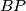$BP$ and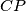$CP$ meet again its circumcircle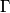$\Gamma$ at$K$,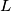$L$, respectively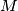$M$. The tangent line at$C$ to$\Gamma$ meets the line$AB$ at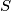$S$. Show that from$SC = SP$ follows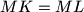$MK = ML$.

Proposed by Marcin E. Kuczma, Poland